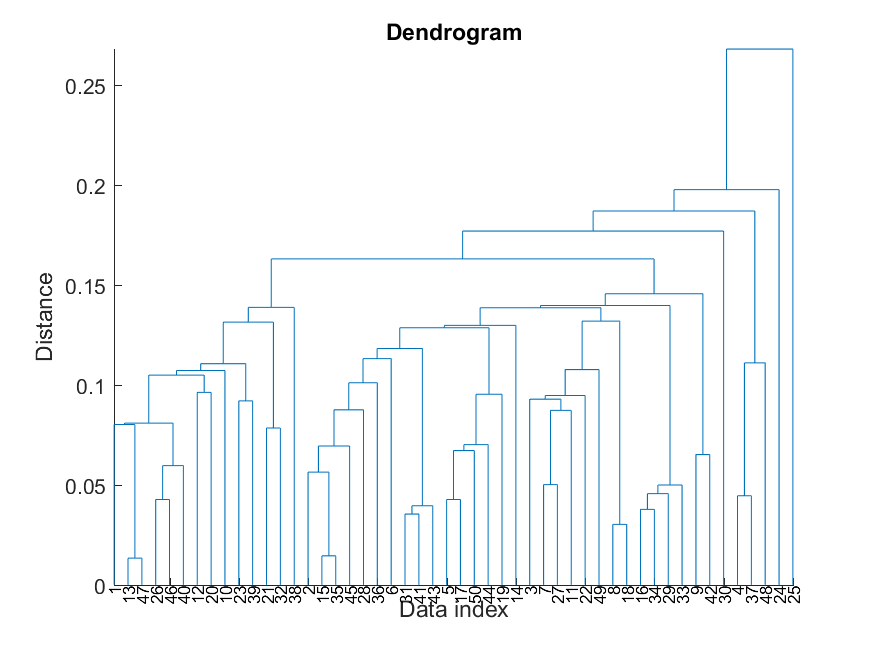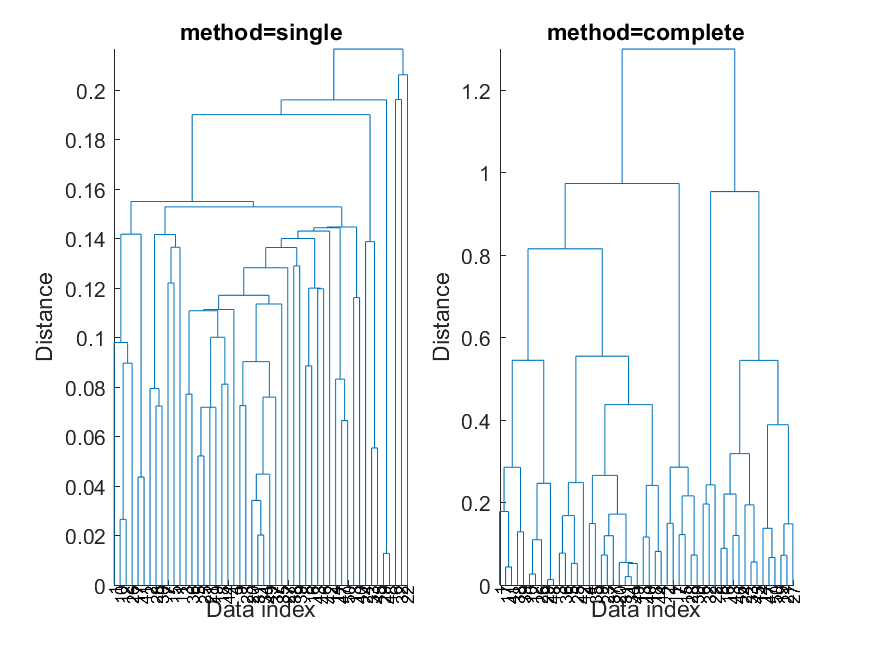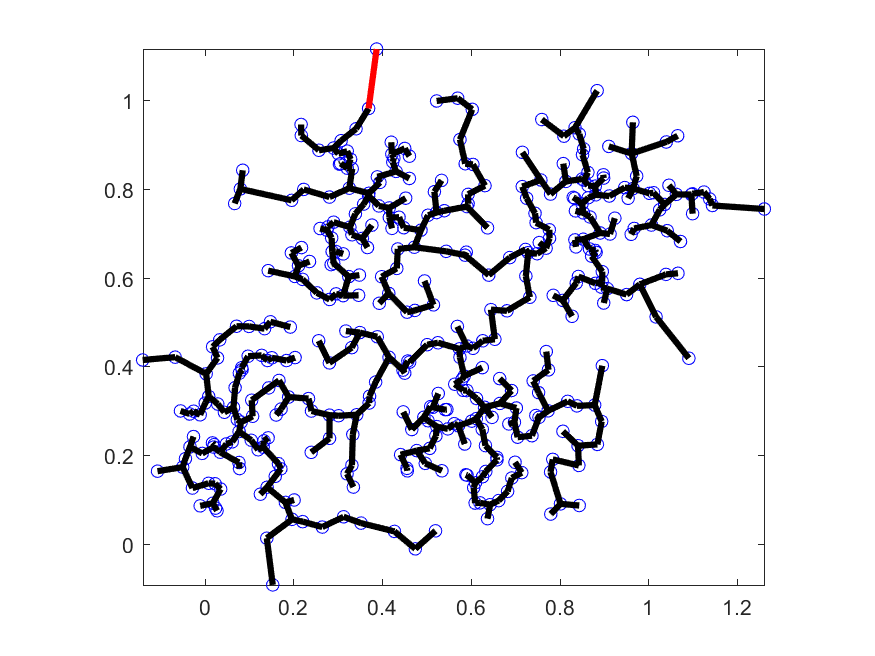## 3-2 Hierarchical Clustering (?Žĺ±¤ĺĽŹĺ?çľ¤ć?)

[chinese][all]

Hierarchical clustering tries to combine or divide a dataset into clusters iteratively, such that a tree-like hierarchical structure is created, primarily for data visualization. To construct such a hierarchical tree structure, we can adopt two approaches:

• Agglomerative hierarchical clustering: This is a bottom-up approach. We can start from the tree leaves (or data instances) and combine two nearest clusters into one at each iteration.
• Divisive hierarchical clustering: This is a top-down approach. We can start from the root of the tree (which contains all data instances) and select a cluster to split at each iteration.
We shall focus on the agglomerative (bottom-up) hierarchical clustering in this section.

For agglomerative hierarchical clustering, we start with the leaves of the tree and move up. In other words, if we have a dataset of size n, then we have n clusters at the beginning, with each data point being a cluster. Then we merge clusters iteratively to move up the tree structure. The algorithm is described next.

1. At the beginning, each data point is a cluster denoted by $C_i, i=1, 2, \dots, n$.
2. Find the nearest two clusters $C_i$ and $C_j$ within all clusters.
3. Combine $C_i$ and $C_j$ into a new cluster.
4. If the number of clusters is equal to the desired one, stop. Otherwise go back to step 2 to create more clusters.
The following example demonstrates the dendrogram after agglomerative hierarchical clustering.

Example 1: hierClusteringPlot01.mdata=rand(2, 50); % 50 data instances of dim 2 distMat=distPairwise(data); % Distance matrix of 50 by 50 hcOutput=hierClustering(distMat); hierClusteringPlot(hcOutput); % Plot the dendrogramIn order to make the algorithm more concrete, We need to define what is meant by "the nearest two clusters". In fact there are several distance functions to compute the distance between two clusters. Different cluster distance functions result in different tree structures. Commonly used cluster distance functions are listed next.

• Single-linkage agglomerative algorithm: The distance between two clusters is the the shortest distance between members of these two clusters: $$d(C_i, C_j)=\min_{\mathbf{a}\in C_i, \mathbf{b}\in C_j} d(\mathbf{a}, \mathbf{b})$$
• Complete-linkage agglomerative algorithm: The distance between two clusters is the longest distance between members of these two clusters: $$d(C_i, C_j)=\max_{\mathbf{a}\in C_i, \mathbf{b}\in C_j} d(\mathbf{a}, \mathbf{b})$$
• Average-linkage agglomerative algorithm: The distance between two clusters is the average distnace between members of these two clusters: $$d(C_i, C_j)=\sum_{\mathbf{a}\in C_i, \mathbf{b}\in C_j} \frac{d(\mathbf{a}, \mathbf{b})}{|C_i||C_j|},$$ where $|C_i|$ and $|C_j|$ are the sizes for $C_i$ and $C_j$, respectively.
• Ward's method: The distance between two clusters is defined as the sum of the squared distance to the mean of the combined clusters: $$d(C_i, C_j)=\sum_{\mathbf{a}\in C_i \cup C_j} \|\mathbf{a}-\mathbf{\mu}\|,$$ where $\mathbf{\mu}$ is the mean vector of $C_i \cup C_j$.

We apply different cluster distance functions to obtain the corresponding tree structures, as follows:

Example 2: hierClusteringPlot02.mdata=rand(2, 50); % 50 data instances of dim 2 distMat=distPairwise(data); % Distance matrix of 50 by 50 method='single'; hcOutput=hierClustering(distMat, method); subplot(1,2,1); hierClusteringPlot(hcOutput); title(['method=', method]); method='complete'; hcOutput=hierClustering(distMat, method); subplot(1,2,2); hierClusteringPlot(hcOutput); title(['method=', method]);From the resultant tree structures, we can observe the following trends:

• Single linkage tends to make the tree slide to one side, with bigger clusters stay big and smaller clusters stay small.
• Complete linkage tends to generate balanced trees, with all clusters growing big simultaneously.
If you want to see the animation of the clustering process, try the next example:

Example 3: hierClusteringAnim01.mdata=dcData(6); data=data.input; dataNum=size(data,2); distMat=distPairwise(data, data); distMat(1:dataNum+1:dataNum^2)=inf; % Diagonal elements should always be inf. method='single'; % 'single' or 'complete' level=hierClustering(distMat, method); hierClusteringAnim(data, distMat, level);It can be proved that the resultant connections via single linkage over a 2D dataset is actually the minimum spanning tree of the dataset.

Data Clustering and Pattern Recognition (¸ę®Ć¤Ŕ¸s»PĽË¦ˇżë»{)